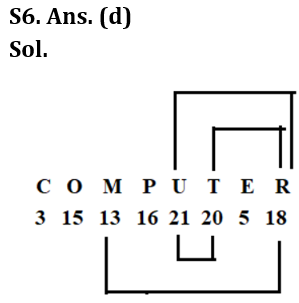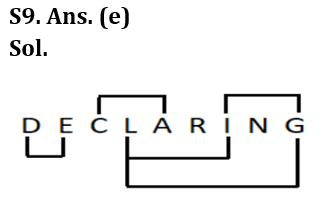Latest Banking jobs   »

# Reasoning Ability Quiz For Bank Foundation 2023-27th February

Q1. What will come in place of question mark (?) from left to right respectively to make the expressions A ≤ D and E > H definitely true?
A ≤ B = C ? D < E >F ? G ≥ H
(a) =, <
(b) <, =
(c) ≤, ≥
(d) >, ≥
(e) None of these

Q2. What will come in place of question mark (?)from left to right respectively to make the expressions P ≥ S and S < U definitely true?
P ? Q = R ≥ S ? T < U = V
(a) ≥, ≤
(b) <, =
(c) =, ≥
(d) >, ≥
(e) None of these

Direction (3-5): In the following questions assuming the given statement to be true, find which of the conclusion(s) among given conclusions is/are definitely true and then give your answers accordingly.

Q3. Statements: X ≤ C ≥ S > D, R ≤ T = X, Q = E ≥ R
Conclusions: I. R = C
II. R < C
(a) Only I is true
(b) Only II is true
(c) Either I or II is true
(d) Neither I nor II is true
(e) Both I and II are true

Q4. Statements: R ≥ C ≤ V, T ≥ S = R, V = E ≤ Y = X
Conclusions: I. C < X
II. C = X
(a) Only I is true
(b) Only II is true
(c) Either I or II is true
(d) Neither I nor II is true
(e) Both I and II are true

Q5. Statements: U ≤ R < T, G = H > Y ≤ U, T = C > V
Conclusions: I. G > R
II. Y < C
(a) Only I is true
(b) Only II is true
(c) Either I or II is true
(d) Neither I nor II is true
(e) Both I and II are true

Q6. How many such pairs of letters are there in the word “COMPUTER” each of which has as many letters between them in the word (in both forward and backward directions) as in the English alphabet?
(a) One
(b) Two
(c) Three
(d) Four
(e) More than four

Q7. How many digits in the number “893642” retain their position if they are rearranged in ascending and descending order both from left to right?
(a) None
(b) One
(c) Two
(d) Three
(e) More than three

Q8. Which letter is the third letter of the meaningful word formed by using the letters which are at third, fourth, sixth and seventh place from the left end in the word ‘JUSTICE’? If more than one word is formed, then select ‘X ‘ as your answer. If no word is formed, select ‘Y’ as your answer?
(a) E
(b) X
(c) Y
(d) T
(e) C

Q9. How many such pairs of letters are there in the word ‘DECLARING’ each of which has as many letters between them in the word as in the English alphabet (both forward and backward)?
(a) None
(b) One
(c) Two
(d) Three
(e) More than three

Q10. If in the word “TRANSDUCE”, all the vowels are changed into the immediate next letter and all the consonants are changed into the immediate previous letter according to the English alphabetical series, then which letter will appear more than once?
(a) S
(b) B
(c) F
(d) C
(e) None

Direction (11-15): Study the information alphanumeric symbol series given below and answer the questions based on it.

A 5 @ D G # 2 J % \$ E G N 1 7 @ & L J 5 1 @ 6 K P & 2 \$ 4 V 5 6 @ 2 S 5 & M 6 P

Q11. Which of the following element will be sixth from the left end when all the symbols are dropped and then starting thirteen elements are reversed?
(a) E
(b) N
(c) G
(d) 5
(e) None of these

Q12. How many symbols are there in the above series which are immediately preceded by a digit and immediately followed by a letter?
(a) One
(b) Two
(c) Three
(d) Four
(e) More than four

Q13. Which element is fourth to the left of the element which is eighth to the right of the one which is third from the extreme left end of the series?
(a) #
(b) @
(c) 2
(d) 7
(e) None of these

Q14. In the series, if the number 5 is inserted after every fifth element when counts from the left end then how many pairs are there in the series in which a letter is immediately followed by 5?
(a) Less than five
(b) Five
(c) Six
(d) More than six
(e) None of these

Q15. If all the even numbers and vowels are dropped from the series and then the order of elements in the series gets reversed, which of the following element is sixth from the left end and ninth from the right end of the arrangement respectively?
(a) ’ @ ’ and ‘G’
(b) ‘G’ and ‘6’
(c) ‘S’ and ‘N’
(d) ‘5’ and ‘\$’
(e) None of these

Solutions

S1. Ans. (c)
Sol. To make the expressions A ≤ D and E > H definitely true,
A ≤ B = C ≤ D < E > F ≥ G ≥ H
Clearly, ≤, ≥ will come in place of question mark (?).

S2. Ans. (a)
Sol. To make the expressions P ≥ S and S < U definitely true,
P ≥ Q = R ≥ S ≤ T < U = V
Clearly, ≥, ≤ will come in place of question mark (?).

Direction (3-5):
S3. Ans. (c)
Sol. Conclusions: I. R = C – False
II. R < C – False
Hence, either conclusion I or II follows.

S4. Ans. (c)
Sol. Conclusions: I. C < X – False
II. C = X – False
Hence, either conclusion I or II follows.

S5. Ans.(b):
Sol. Conclusions: I. G > R – False
II. Y < C – TrueS7. Ans. (c)
Sol. Let we arrange “893642” in ascending order we get: 234689
Hence we observe that one number 6 retain its position when “893642” is rearranged in ascending order.
Let we arrange “893642” in descending order we get: 986432
Hence we observe that one number 2 retain its position when “893642” is rearranged in descending order.

S8. Ans. (e)
Sol. Meaning full word = sect
So, third letter of the meaningful word is C.S10. Ans.(b)
Sol. TRANSDUCE – SQBMRCVBF
Hence, option (b) is the correct answer.

Solution (11-15):
S11. Ans. (c)
Sol. If all the symbols are dropped, we will get:
A 5 D G 2 J E G N 1 7 L J 5 1 6 K P 2 4 V 5 6 2 S 5 M 6 P
Now, if starting thirteen elements are reversed, we will get:
J L 7 1 N G E J 2 G D 5 A 5 1 6 K P 2 4 V 5 6 2 S 5 M 6 P
Now, ‘G’ will be sixth from the left end.

S12. Ans. (b)
Sol. Two symbols are there which are immediately preceded by a digit and immediately followed by a letter =@ and &
A 5 @ D G # 2 J % \$ E G N 1 7 @ & L J 5 1 @ 6 K P & 2 \$ 4 V 5 6 @ 2 S 5 & M 6 P

S13. Ans. (c)
Sol. Third element from the left end = @
“E” is 8th to the right of “@”.
“2” is 4th to the left of “E”.

S14. Ans. (d)
Sol. If number 5 is inserted after every fifth element we will get seven letters which are immediately followed by 5.
A 5 @ D G 5 # 2 J % \$ 5 E G N 1 7 5 @ & L J 5 5 1 @ 6 K P5 & 2 \$ 4 V5 5 6 @ 2 S 5 5 & M 6 P 5

S15. Ans. (a)
Sol. Now, ‘@’ is the sixth element from the left end of the arrangement. ‘G’ is the ninth element from the right end of the arrangement.## FAQs

### What is the selection process of the Bank Clerk?

The selection process of the Bank Clerk is Prelims & Mains.

#### Congratulations!Union Budget 2023-24: Free PDF